# sandbox.rng_mrg – MRG random number generator¶

## API¶

Implementation of MRG31k3p random number generator for Aesara.

Generator code in SSJ package (L’Ecuyer & Simard). http://www.iro.umontreal.ca/~simardr/ssj/indexe.html

The MRG31k3p algorithm was published in:

1. L’Ecuyer and R. Touzin, Fast Combined Multiple Recursive Generators with Multipliers of the form a = +/- 2^d +/- 2^e, Proceedings of the 2000 Winter Simulation Conference, Dec. 2000, 683-689.

The conception of the multi-stream from MRG31k3p was published in:

1. L’Ecuyer and R. Simard and E. Jack Chen and W. David Kelton, An Object-Oriented Random-Number Package with Many Long Streams and Substreams, Operations Research, volume 50, number 6, 2002, 1073-1075.
class aesara.sandbox.rng_mrg.DotModulo[source]

Efficient and numerically stable implementation of a dot product followed by a modulo operation. This performs the same function as matVecModM.

We do this 2 times on 2 triple inputs and concatenating the output.

c_code(node, name, inputs, outputs, sub)[source]

Return the C implementation of an Op.

Returns C code that does the computation associated to this Op, given names for the inputs and outputs.

Parameters: node (Apply instance) – The node for which we are compiling the current C code. The same Op may be used in more than one node. name (str) – A name that is automatically assigned and guaranteed to be unique. inputs (list of strings) – There is a string for each input of the function, and the string is the name of a C variable pointing to that input. The type of the variable depends on the declared type of the input. There is a corresponding python variable that can be accessed by prepending "py_" to the name in the list. outputs (list of strings) – Each string is the name of a C variable where the Op should store its output. The type depends on the declared type of the output. There is a corresponding Python variable that can be accessed by prepending "py_" to the name in the list. In some cases the outputs will be preallocated and the value of the variable may be pre-filled. The value for an unallocated output is type-dependent. sub (dict of strings) – Extra symbols defined in CLinker sub symbols (such as 'fail').
c_code_cache_version()[source]

Return a tuple of integers indicating the version of this Op.

An empty tuple indicates an “unversioned” Op that will not be cached between processes.

The cache mechanism may erase cached modules that have been superseded by newer versions. See ModuleCache for details.

c_code_cache_version_apply

make_node(A, s, m, A2, s2, m2)[source]

Construct an Apply node that represent the application of this operation to the given inputs.

This must be implemented by sub-classes.

Returns: node – The constructed Apply node. Apply
perform(node, inputs, outputs)[source]

Calculate the function on the inputs and put the variables in the output storage.

Parameters: node – The symbolic Apply node that represents this computation. inputs – Immutable sequence of non-symbolic/numeric inputs. These are the values of each Variable in node.inputs. output_storage – List of mutable single-element lists (do not change the length of these lists). Each sub-list corresponds to value of each Variable in node.outputs. The primary purpose of this method is to set the values of these sub-lists. params – A tuple containing the values of each entry in Op.__props__.

Notes

The output_storage list might contain data. If an element of output_storage is not None, it has to be of the right type, for instance, for a TensorVariable, it has to be a NumPy ndarray with the right number of dimensions and the correct dtype. Its shape and stride pattern can be arbitrary. It is not guaranteed that such pre-set values were produced by a previous call to this Op.perform(); they could’ve been allocated by another Op’s perform method. An Op is free to reuse output_storage as it sees fit, or to discard it and allocate new memory.

class aesara.sandbox.rng_mrg.MRG_RandomStream(seed=12345)[source]

Module component with similar interface to numpy.random (numpy.random.RandomState).

Parameters: seed (int or list of 6 int) – A default seed to initialize the random state. If a single int is given, it will be replicated 6 times. The first 3 values of the seed must all be less than M1 = 2147483647, and not all 0; and the last 3 values must all be less than M2 = 2147462579, and not all 0.
choice(size=1, a=None, replace=True, p=None, ndim=None, dtype='int64', nstreams=None, **kwargs)[source]

Sample size times from a multinomial distribution defined by probabilities p, and returns the indices of the sampled elements. Sampled values are between 0 and p.shape-1. Only sampling without replacement is implemented for now.

Parameters: size (integer or integer tensor (default 1)) – The number of samples. It should be between 1 and p.shape-1. a (int or None (default None)) – For now, a should be None. This function will sample values between 0 and p.shape-1. When a != None will be implemented, if a is a scalar, the samples are drawn from the range 0,…,a-1. We default to 2 as to have the same interface as RandomStream. replace (bool (default True)) – Whether the sample is with or without replacement. Only replace=False is implemented for now. p (2d numpy array or aesara tensor) – the probabilities of the distribution, corresponding to values 0 to p.shape-1. Example (p = [[.98, .01, .01], [.01, .49, .50]] and size=1 will) – [ (probably result in) – size=2 (]. When setting) – this – [[0 (will probably result in) – 1] – [2 – 1]]. –

Notes

-ndim is only there keep the same signature as other uniform, binomial, normal, etc.

-Does not do any value checking on pvals, i.e. there is no check that the elements are non-negative, less than 1, or sum to 1. passing pvals = [[-2., 2.]] will result in sampling [[0, 0]]

-Only replace=False is implemented for now.

get_substream_rstates(n_streams, dtype, inc_rstate=True)[source]

Initialize a matrix in which each row is a MRG stream state, and they are spaced by 2**72 samples.

inc_rstate()[source]

Update self.rstate to be skipped 2^134 steps forward to the next stream start.

multinomial(size=None, n=1, pvals=None, ndim=None, dtype='int64', nstreams=None, **kwargs)[source]

Sample n (n needs to be >= 1, default 1) times from a multinomial distribution defined by probabilities pvals.

Example : pvals = [[.98, .01, .01], [.01, .49, .50]] and n=1 will probably result in [[1,0,0],[0,0,1]]. When setting n=2, this will probably result in [[2,0,0],[0,1,1]].

Notes

-size and ndim are only there keep the same signature as other uniform, binomial, normal, etc. TODO : adapt multinomial to take that into account

-Does not do any value checking on pvals, i.e. there is no check that the elements are non-negative, less than 1, or sum to 1. passing pvals = [[-2., 2.]] will result in sampling [[0, 0]]

normal(size, avg=0.0, std=1.0, ndim=None, dtype=None, nstreams=None, truncate=False, **kwargs)[source]

Sample a tensor of values from a normal distribution.

Parameters: size (int_vector_like) – Array dimensions for the output tensor. avg (float_like, optional) – The mean value for the truncated normal to sample from (defaults to 0.0). std (float_like, optional) – The standard deviation for the truncated normal to sample from (defaults to 1.0). truncate (bool, optional) – Truncates the normal distribution at 2 standard deviations if True (defaults to False). When this flag is set, the standard deviation of the result will be less than the one specified. ndim (int, optional) – The number of dimensions for the output tensor (defaults to None). This argument is necessary if the size argument is ambiguous on the number of dimensions. dtype (str, optional) – The data-type for the output tensor. If not specified, the dtype is inferred from avg and std, but it is at least as precise as floatX. kwargs – Other keyword arguments for random number generation (see uniform). samples – A Aesara tensor of samples randomly drawn from a normal distribution. TensorVariable
seed(seed=None)[source]

Re-initialize each random stream.

Parameters: seed (None or integer in range 0 to 2**30) – Each random stream will be assigned a unique state that depends deterministically on this value. None
truncated_normal(size, avg=0.0, std=1.0, ndim=None, dtype=None, nstreams=None, **kwargs)[source]

Sample a tensor of values from a symmetrically truncated normal distribution.

Parameters: size (int_vector_like) – Array dimensions for the output tensor. avg (float_like, optional) – The mean value for the truncated normal to sample from (defaults to 0.0). std (float_like, optional) – The standard deviation for the truncated normal to sample from (defaults to 1.0). ndim (int, optional) – The number of dimensions for the output tensor (defaults to None). This argument is necessary if the size argument is ambiguous on the number of dimensions. dtype (str, optional) – The data-type for the output tensor. If not specified, the dtype is inferred from avg and std, but it is at least as precise as floatX. kwargs – Other keyword arguments for random number generation (see uniform). samples – A Aesara tensor of samples randomly drawn from a truncated normal distribution. TensorVariable
uniform(size, low=0.0, high=1.0, ndim=None, dtype=None, nstreams=None, **kwargs)[source]

Sample a tensor of given size whose element from a uniform distribution between low and high.

If the size argument is ambiguous on the number of dimensions, ndim may be a plain integer to supplement the missing information.

Parameters: low – Lower bound of the interval on which values are sampled. If the dtype arg is provided, low will be cast into dtype. This bound is excluded. high – Higher bound of the interval on which values are sampled. If the dtype arg is provided, high will be cast into dtype. This bound is excluded. size – Can be a list of integer or Aesara variable (ex: the shape of other Aesara Variable). dtype – The output data type. If dtype is not specified, it will be inferred from the dtype of low and high, but will be at least as precise as floatX.
aesara.sandbox.rng_mrg.guess_n_streams(size, warn=False)[source]

Return a guess at a good number of streams.

Parameters: warn (bool, optional) – If True, warn when a guess cannot be made (in which case we return 60 * 256).
class aesara.sandbox.rng_mrg.mrg_uniform(output_type, inplace=False)[source]
c_code(node, name, inp, out, sub)[source]

Return the C implementation of an Op.

Returns C code that does the computation associated to this Op, given names for the inputs and outputs.

Parameters: node (Apply instance) – The node for which we are compiling the current C code. The same Op may be used in more than one node. name (str) – A name that is automatically assigned and guaranteed to be unique. inputs (list of strings) – There is a string for each input of the function, and the string is the name of a C variable pointing to that input. The type of the variable depends on the declared type of the input. There is a corresponding python variable that can be accessed by prepending "py_" to the name in the list. outputs (list of strings) – Each string is the name of a C variable where the Op should store its output. The type depends on the declared type of the output. There is a corresponding Python variable that can be accessed by prepending "py_" to the name in the list. In some cases the outputs will be preallocated and the value of the variable may be pre-filled. The value for an unallocated output is type-dependent. sub (dict of strings) – Extra symbols defined in CLinker sub symbols (such as 'fail').
c_code_cache_version()[source]

Return a tuple of integers indicating the version of this Op.

An empty tuple indicates an “unversioned” Op that will not be cached between processes.

The cache mechanism may erase cached modules that have been superseded by newer versions. See ModuleCache for details.

c_code_cache_version_apply

c_support_code(**kwargs)[source]

Return utility code for use by a Variable or Op.

This is included at global scope prior to the rest of the code for this class.

Question: How many times will this support code be emitted for a graph with many instances of the same type?

Return type: str
make_node(rstate, size)[source]

Construct an Apply node that represent the application of this operation to the given inputs.

This must be implemented by sub-classes.

Returns: node – The constructed Apply node. Apply
perform(node, inp, out, params)[source]

Calculate the function on the inputs and put the variables in the output storage.

Parameters: node – The symbolic Apply node that represents this computation. inputs – Immutable sequence of non-symbolic/numeric inputs. These are the values of each Variable in node.inputs. output_storage – List of mutable single-element lists (do not change the length of these lists). Each sub-list corresponds to value of each Variable in node.outputs. The primary purpose of this method is to set the values of these sub-lists. params – A tuple containing the values of each entry in Op.__props__.

Notes

The output_storage list might contain data. If an element of output_storage is not None, it has to be of the right type, for instance, for a TensorVariable, it has to be a NumPy ndarray with the right number of dimensions and the correct dtype. Its shape and stride pattern can be arbitrary. It is not guaranteed that such pre-set values were produced by a previous call to this Op.perform(); they could’ve been allocated by another Op’s perform method. An Op is free to reuse output_storage as it sees fit, or to discard it and allocate new memory.

class aesara.sandbox.rng_mrg.mrg_uniform_base(output_type, inplace=False)[source]
R_op(inputs, eval_points)[source]

Construct a graph for the R-operator.

This method is primarily used by Rop.

Parameters: inputs – The Op inputs. eval_points – A Variable or list of Variables with the same length as inputs. Each element of eval_points specifies the value of the corresponding input at the point where the R-operator is to be evaluated. rval[i] should be Rop(f=f_i(inputs), wrt=inputs, eval_points=eval_points).
grad(inputs, ograd)[source]

Construct a graph for the gradient with respect to each input variable.

Each returned Variable represents the gradient with respect to that input computed based on the symbolic gradients with respect to each output. If the output is not differentiable with respect to an input, then this method should return an instance of type NullType for that input.

Using the reverse-mode AD characterization given in _, for a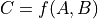representing the function implemented by the Op and its two arguments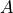and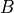, given by the Variables in inputs, the values returned by Op.grad represent the quantities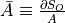and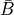, for some scalar output term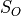of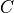in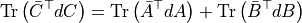Parameters: inputs – The input variables. output_grads – The gradients of the output variables. grads – The gradients with respect to each Variable in inputs. ..  Giles, Mike. 2008. “An Extended Collection of Matrix Derivative Results for Forward and Reverse Mode Automatic Differentiation.”
aesara.sandbox.rng_mrg.multMatVect(v, A, m1, B, m2)[source]

Multiply the first half of v by A with a modulo of m1 and the second half by B with a modulo of m2.

Notes

The parameters of dot_modulo are passed implicitly because passing them explicitly takes more time than running the function’s C-code.